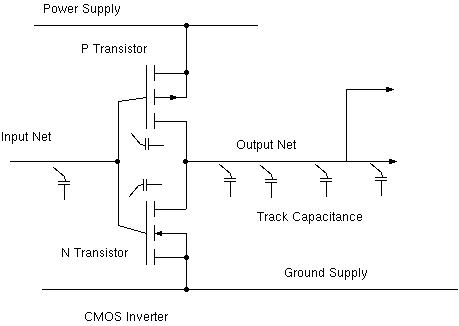# Power Estimation Formula

Power is measured in Watts and P = V x I = E x f

Gate current I = Static Current (leakage) + Dynamic Current.

Early CMOS (VCC 5 volts): negligible static current,

but today at VCC of 1.3 volts it's 30 % of consumption.

Dynamic current = Short circuit current + Dynamic charge current.
 Dynamic charge current computation: All energy in a net/gates is wasted each time it goes from one to zero. The energy in a capacitor is E = CV^2/2. Dominant capacitance is proportional to net length. Gate input and output capacitance also contribute to C. Activity ratio, a: percentage of clock cycles that see a transition. The net toggle rate = Operating frequency of the chip (f) \times ~ a. For leakage and short circuit power see cell library.(C) 2008-10, DJ Greaves, University of Cambridge, Computer Laboratory.